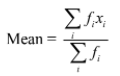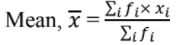# RS Aggarwal Solutions Chapter 9 Mean, Mode and Median Exercise 9D Class 10 Maths

 Chapter Name RS Aggarwal Chapter 9 Mean, Mode and Median Book Name RS Aggarwal Mathematics for Class 10 Other Exercises Exercise 9AExercise 9BExercise 9CExercise 9EExercise 9F Related Study NCERT Solutions for Class 10 Maths

### Exercise 9D Solutions

1. Find the mean, median and mode of the following data:

 Class 0 - 10 10 - 20 20 - 30 30 - 40 40 - 50 50 - 60 60 - 70 Frequency 4 4 7 10 12 8 5

Solution

To find the mean let us put the data in the table given below:

 Class Frequency (fi) Class mark (xi) fi xi 0 - 10 4 5 20 10 - 20 4 15 60 20 - 30 7 25 175 30 - 40 10 35 350 40 - 50 12 45 540 50 – 60 8 55 440 60 70 5 65 325 Total Σfi = 50 Σfi xi = 1910

= 1910/50

= 38.2

Thus, the mean of the given data is 38.2.

Now, to find the median let us put the data in the table given below:

 Class Frequency (fi) Cumulative Frequency (cf) 0 - 10 4 4 10 - 20 4 8 20 - 30 7 15 30 - 40 10 25 40 - 50 12 37 50 – 60 8 45 60 – 70 5 50 Total N = Σfi = 50

Now, N = 50

⇒ N/2 = 25

The cumulative frequency just greater than 25 is 37 and the corresponding class is 40 – 50.

Thus, the median class is 40 – 50.

∴ l = 40, h = 10, N = 50, f = 12 and cf = 25.

Now,

Median = l + ((N/2 – cf)/f) × h

= 40 + (25 – 25)/12 × 10

= 40.

Thus, the median is 40.

We know that,

Mode = 3(Median) – 2(Mean)

= 3 × 40 – 2 × 38.2

= 120 – 76.4

= 43.6

Hence, Mean = 38.2, Median = 40 and Mode = 43.6

2. Find the mean, median and mode of the following data:

 Class 0 - 20 20 - 40 40 - 60 60 - 80 80 - 100 100 - 120 120 - 140 Frequency 6 8 10 12 6 5 3

Solution

To find the mean let us put the data in the table given below:

 Class Frequency (fi) Class mark (xi) fi xi 0 - 20 6 10 60 20 - 40 8 30 240 40 - 60 10 50 500 60 - 80 12 70 840 80 - 100 6 90 540 100 - 120 5 110 550 120 - 140 3 130 390 Total Σfi = 50 Σfi xi = 3120

= 3120/50

= 62.4

Thus, the mean of the given data is 62.4.

Now, to find the median let us put the data in the table given below:

 Class Frequency (fi) Cumulative Frequency (cf) 0 - 20 6 6 20 - 40 8 14 40 - 60 10 24 60 - 80 12 36 80 - 100 6 42 100 – 120 5 47 120 – 140 3 50 Total N = Σfi = 50

Now, N = 50

⇒ N/2 = 25

The cumulative frequency just greater than 25 is 36 and the corresponding class is 60 – 80.

Thus, the median class is 60 – 80.

∴ l = 60, h = 20, N = 50, f = 12 and cf = 24.

Now,

Median = l + ((N/2 – cf)/f) × h

= 60 + (25 – 24)/12 × 20

= 60 + 1.67

= 61.67

Thus, the median is 61.67.

We know that,

Mode = 3(Median) – 2(mean)

= 3 × 61.67 – 2 × 62.4

= 185.01 – 124.8

= 60.21

Hence, Mean = 62.4, Median = 61.67 and Mode = 60.21

3. Find the mean, median and mode of the following data:

 Class 0 - 50 5 - 100 100 - 150 150 - 200 200 - 250 250 - 300 300 - 350 Frequency 2 3 5 6 5 3 1

Solution

To find the mean let us put the data in the table given below:

 Class Frequency (fi) Class mark (xi) fi xi 0 - 50 2 25 50 50 - 100 3 75 225 100 - 150 5 125 625 150 - 200 6 175 1050 200 - 250 5 225 1125 250 - 300 3 275 825 300 – 350 1 325 325 Total Σfi  = 25 Σfi xi = 4225

= 4225/25

= 169

Thus, mean of the given data is 169.

Now, to find the median let us put the data in the table given below:

 Class Frequency (fi) Cumulative frequency (cf) 0 - 50 2 2 50 - 100 3 5 100 - 150 5 10 150 - 200 6 16 200 – 250 5 21 250 – 300 3 24 300 – 350 1 25 Total N = Σfi = 25

Now, N = 25, ⇒ N/2 = 12.5

The cumulative frequency just greater than 12.5 is 16 and the corresponding class is 150 – 200.

Thus, the median class is 150 – 200.

∴ l = 150, h = 50, N = 25, f = 6 and cf = 10.

Now,

Median = l + ((N/2 – cf)/f) × h

= 150 + (12.5 – 10)/6 × 50

= 150 + 20.83

= 170.83

Thus, the median is 170.83

We know that,

Mode = 3(Median) – 2(mean)

= 3 × 170.83 – 2 × 169

= 512.49 – 33.8

= 174.49

Hence, mean = 169, Median = 170.83 and Mode = 174.49

4. Find the mean, median and mode of the following data:

 Marks Obtained 25 - 35 35 - 45 45 - 55 55 - 65 65 - 75 75 - 85 No. of students 7 31 33 17 11 1

Solution

To find the mean let us put the data in the table given below:

 Marks Obtained Number of students (fi) Class mark (xi) fi xi 25 - 35 7 30 210 35 - 45 31 40 1240 45 - 55 33 50 1650 55 - 65 17 60 1020 65 - 75 11 70 770 75 - 85 1 80 80 Total Σfi = 100 Σfi xi = 4970= 4970/100

= 49.7

Thus, mean of the given data is 49.7.

Now, to find the median let us put the data in the table given below:

 Class Frequency (fi) Cumulative frequency (cf) 25 - 35 7 7 35 - 45 31 38 45 - 55 33 71 55 - 65 17 88 65 - 75 11 99 75 - 85 1 100 Total N = Σfi = 100

Now, N = 100 ⇒ N/2 = 50.

The cumulative frequency just greater than 50 is 71 and the corresponding class is 45 – 55.

Thus, the median class is 45 – 55.

∴ l = 45, h = 10, N = 100, f = 33 and cf = 38.

Now,

Median = l + ((N/2 – cf)/f) × h

= 45 + (50 – 38)/33 × 10

= 45 + 3.64

= 48.64

Thus, the median is 48.64

We know that,

Mode = 3(Median) – 2(mean)

= 3 × 48.64 – 2 × 49.70

= 145.92 – 99.4

= 46.52

Hence, Mean = 49.70, Median = 48.64 and Mode = 46.52

5. A survey regarding the heights (in cm) of 50 girls of a class was conducted and the following data was obtained:

 Height in cm 120 - 130 130 - 140 140 - 150 150 - 160 160 - 170 No. of girls 2 8 12 20 8

Find the mean, median and mode of the above data.

Solution

We have the following:

 Height in cm Mid value (xi) Frequency (fi) Cumulative frequency (fi × xi) 120 - 130 125 2 2 250 130 - 140 135 8 10 1080 140 - 150 145 12 22 1740 150 - 160 155 20 42 3100 160 - 170 165 8 50 1320 Σfi = 50 Σfi × xi = 7490
= 7490/50

= 149.8

Now, N = 50

⇒ N/2 = 25.

The cumulative frequency just greater than 25 is 42 and the corresponding class is 150 – 160.

Thus, the median class is 150 – 160.

∴ l = 150, h = 10, f = 20, c = cf of preceding class=22 and N/2 = 25

Now,

Median, Me = l + {h × ((N/2 – c)/f)}

= 150 + {10 × ((25 – 22)/20)}

= (150 + 10 × 3/20)

= 151.5

Mode = 3(median) – 2(Mean)

= 3 × 151.5 – 2 × 149.8

= 154.9

6. The following table gives the daily income of 50 workers of a factory:

 Daily income (in Rs) 100 - 120 120 - 140 140 - 160 160 - 180 180 - 200 No. of workers 12 14 8 6 10

Find the mean, median and mode of the above data.

Solution

We have the following:

 Daily income Mid values (xi) Frequency (fi) Cumulative frequency (fi × xi) 100 - 120 110 12 12 1320 120 - 140 130 14 26 1820 140 - 160 150 8 34 1200 160 - 180 170 6 40 1020 180 - 200 190 10 50 1900 Σfi = 50 Σfi × xi = 7260

= 7260/50

= 145.2

Now, N = 50

⇒ N/2 = 25

The cumulative frequency just greater than 25 is 26 and the corresponding class is 120 – 140.

Thus, the median class is 120 – 140.

∴ l = 120, h = 20, f = 14, c = cf of preceding class=12 and N/2 = 25

Now,

Median, Me = l + {h × ((N/2 – c)/f)}

= 120 + {20 × (25 – 12)/14}

= (120 + 20 × 13/14)

= 138.57

Mode = 3(median) – 2(mean)

= 3 × 138.57 – 2 × 145.2

= 125.31

7. The table shows the daily expenditure on food of 30 households in a locality:

 Daily expenditure (in Rs) Number of households 100 - 150 6 150 - 200 7 200 - 250 12 250 - 300 3 300 - 350 2

Find the mean and median daily expenditure on food.

Solution

We have the following:

 Daily expenditure (in Rs) Mid value (xi) Frequency (fi) Cumulative frequency (fi × xi) 100 - 150 125 6 6 750 150 - 200 175 7 13 1225 200 - 250 225 12 25 2700 250 - 300 275 3 28 825 300 - 350 325 2 30 650 Σfi = 30 Σfi × xi = 6150= 6150/30

= 205

Now, N = 30

N/2 = 15

The cumulative frequency just greater than 15 is 25 and the corresponding class is 200 – 250.

Thus, the median class is 200 – 250.

l = 200, h = 50, f = 12, c = cf of preceding class = 13 and N/2 = 15

Now,

Median, Me = l + {h × (N/2 – c)/f)}

= 200 + {50 + (15 – 13)/12)}

= (200 + 50 × 2/12)

= 200 + 8.33

= 208.33